# Colleges with the lowest SAT scores in Wisconsin

Top 10 colleges in Wisconsin with the lowest SAT scores
Looking for the colleges with the lowest SAT scores in Wisconsin? Well you're in luck! We've compiled a national college database and have created a list of the top 10 universities with the lowest SAT scores in Wisconsin below. If you are not a good test taker or worried about your test scores, this list is for you. These are the schools whose applicants had the lowest average SAT scores in Wisconsin, which means that you can get into these colleges with a lower SAT score. We also include each college's ACT scores and acceptance rate so that you can see where you would have the easiest time getting in. Read on to find out more.

## Silver Lake College of the Holy Family SAT scores

The average SAT score for Silver Lake College of the Holy Family is 1010.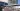The average SAT score of 1010 breaks down into:

• SAT math: 500

The average ACT score for Silver Lake College of the Holy Family is 17 and their acceptance rate is 50%.

## Cardinal Stritch University SAT scores

The average SAT score for Cardinal Stritch University is 1033.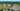The average SAT score of 1033 breaks down into:

• SAT math: 530

The average ACT score for Cardinal Stritch University is 21 and their acceptance rate is 78.3%.

## Alverno College SAT scores

The average SAT score for Alverno College is 1040.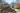The average SAT score of 1040 breaks down into:

• SAT math: 520

The average ACT score for Alverno College is 19 and their acceptance rate is 67.1%.

## University of Wisconsin Stevens Point SAT scores

The average SAT score for University of Wisconsin Stevens Point is 1040.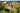The average SAT score of 1040 breaks down into:

• SAT math: 510

The average ACT score for University of Wisconsin Stevens Point is 23 and their acceptance rate is 81%.

## University of Wisconsin Whitewater SAT scores

The average SAT score for University of Wisconsin Whitewater is 1100.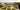The average SAT score of 1100 breaks down into:

• SAT math: 560

The average ACT score for University of Wisconsin Whitewater is 22 and their acceptance rate is 83.2%.

## University of Wisconsin Stout SAT scores

The average SAT score for University of Wisconsin Stout is 1110.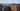The average SAT score of 1110 breaks down into:

• SAT math: 555

The average ACT score for University of Wisconsin Stout is 22 and their acceptance rate is 86%.

## University of Wisconsin Green Bay SAT scores

The average SAT score for University of Wisconsin Green Bay is 1110.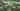The average SAT score of 1110 breaks down into:

• SAT math: 555

The average ACT score for University of Wisconsin Green Bay is 22 and their acceptance rate is 92.5%.

## University of Wisconsin Oshkosh SAT scores

The average SAT score for University of Wisconsin Oshkosh is 1110.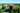The average SAT score of 1110 breaks down into:

• SAT math: 555

The average ACT score for University of Wisconsin Oshkosh is 22 and their acceptance rate is 67.8%.

## University of Wisconsin River Falls SAT scores

The average SAT score for University of Wisconsin River Falls is 1110.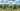The average SAT score of 1110 breaks down into:

• SAT math: 550

The average ACT score for University of Wisconsin River Falls is 23 and their acceptance rate is 75.1%.

## University of Wisconsin Superior SAT scores

The average SAT score for University of Wisconsin Superior is 1110.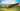The average SAT score of 1110 breaks down into:

• SAT math: 600